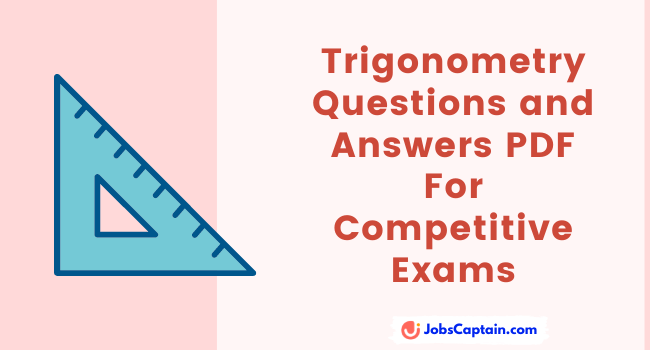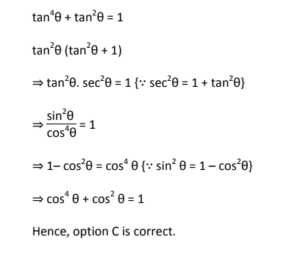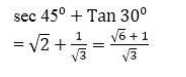# Trigonometry Questions and Answers PDF For Competitive Exams

By | December 20, 2021Below given trigonometry questions and answers PDF Books are very helpful for competitive examinations like SSC, RRB, and Banking.

Trigonometry is the most important topic of quantitative reasoning. These types of questions are frequently asked in the SSC CGL examination.

Question 1: If tan4θ + tan2θ = 1, then the value of cos4θ + cos2θ is ______ .

(A) 8

(B) 10

(C) 1

(D) 2

(C) 1

Explanation:Question 2: (1) Sec 45º + Tan 30º = __________ .

(A) (√6 + 1)/√3

(B)  (1 + √3)/2

(C) (√3 + 2)/√3

(D) 5/√3

(A) (√6 + 1)/√3

Explanation:Practise More on Trigonometry Quiz

Now let’s download trigonometry questions with answer PDF Books for competitive examination in Hindi (हिन्दी) and English.

## Trigonometry Questions PDF

Trigonometry Questions for CDSE, CGL Tier 2, CGL Tier 1, and SSC 10+2 Exams – Download

250+ Trigonometry Previous Year Questions with Solution Free PDF For SSC, RRB, FCI Exams – Download Now

Complete Trigonometry (त्रिकोणमिति) By Gagan Pratap Sir [SSC CGL 2011 to 2011 Questions With Answer Notes PDF] – Download